# The HPIMPUTE Procedure

### Obtaining the Statistics for Imputation

PROC HPIMPUTE first computes the imputation value and then imputes with that value. Some statistics (such as the mean, minimum, and maximum) are computed precisely. The pseudomedian, which is calculated if you specify METHOD=PMEDIAN in the IMPUTE statement, is an estimation of the median. The computation of the median requires sorting the entire data. However, in a distributed computing environment, each grid node contains only a part of the entire data. Sorting all the data in such an environment requires a lot of internode communications, degrading the performance dramatically. To address this challenge, a binning-based method is used to estimate the pseudomedian.

For variable x, assume that the data set is {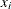}, where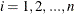. Let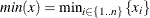, and let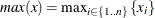. The range of the variable is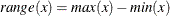.

A simple bucket binning method is used to obtain the basic information. Let
be the number of buckets, ranging from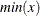to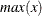. For each bucket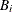,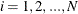, PROC HPIMPUTE keeps following information:

•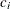: count ofin•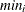: minimum value ofin•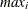: maximum value ofinFor each bucket, the range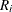is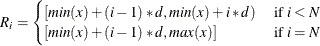where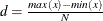To calculate the pseudomedian, PROC HPIMPUTE finds the smallest, such that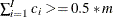, whereis the number of nonmissing observations ofin the data set. Therefore, the pseudomedian value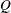is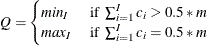is set to 10,000 in PROC HPIMPUTE. Experiments show that the pseudomedian is a good estimate of the median and that the performance is satisfactory.